1625776440

# ROC Curve and AUC. Simple Explanation (with Example)

ROC and AUC curves with simple example are explained at this video which dedicated to all data scientists, and to all Machine Learning specialists.

Explanation is divided into following parts:

• How do we use prediction threshold (cut-off line).
• How do we plot data on Logistic Regression (Sigmoid) curve
• How do we understand Confusion Matrixes (True Positives, True Negatives, False Negatives, and False Positives), Supervised Learning approach.
• How we calculate TPR (True Positive Rate) and how do we use it in plotting ROC.
• How we calculate FPR (False Positive Rate) and how do we use it in plotting ROC (Receiver operating characteristic).
• How we draw AUC (Area Under the Curve) based on ROC curves.
• How we can compare different Machine Learning Binary Classification models and algorithms with AUC.

For get benefit of this video you do not need to know Python or R. Just be careful about main definition of statistics, calculus and go ahead.

I hoped to create one of the best explainable video on Youtube about ROC and AUC. Comment bellow and drop your opinion!

#roccurve
#auccurve
#machinelearningtutorial

#roccurve #auccurve #machinelearningtutorial

## Buddha Community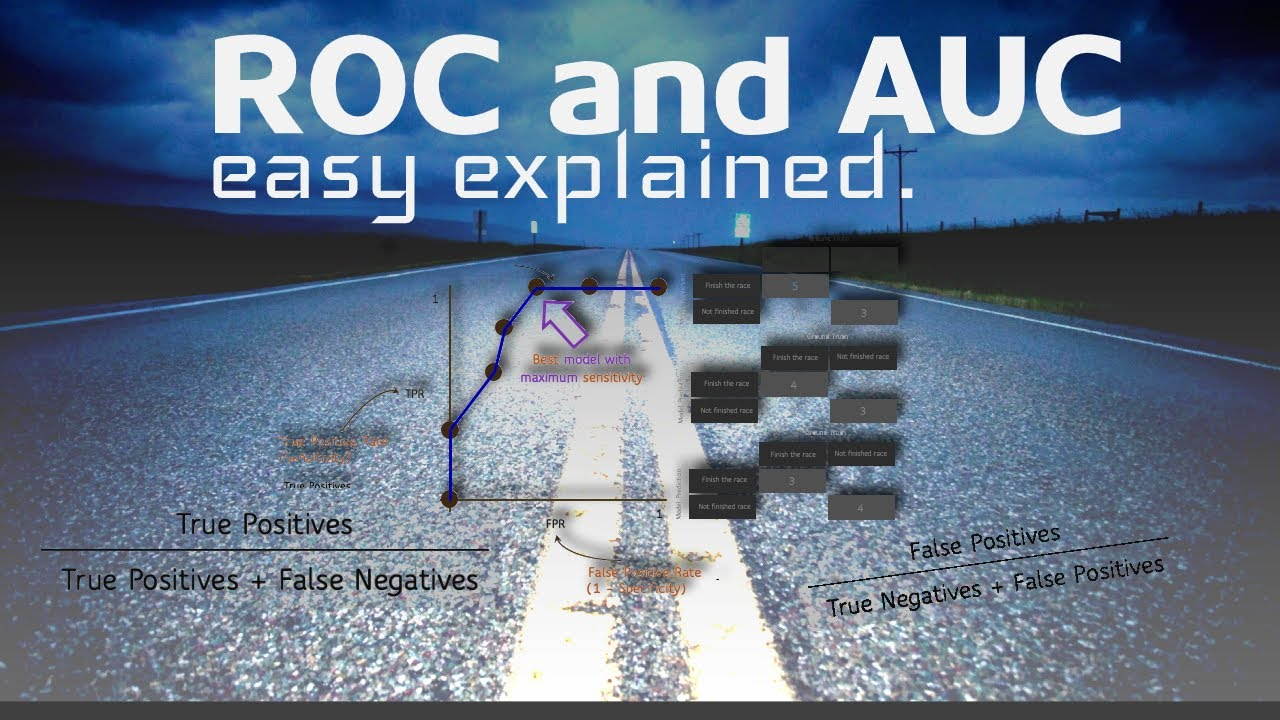1597025340

## ROC Curve and AUC — Detailed understanding and R pROC Package

The world is facing a unique crisis these days and we all are stuck in a never seen before lockdown. As all of us are utilizing this time in many productive ways, I thought of creating some blogs of data concepts I know, not only to share it with the community but also to develop a more deep understanding of the concept as I write it down.

The first one is here about the most loved evaluation metric — The ROC curve.

ROC (Receiver Operating Characteristic) Curve is a way to visualize the performance of a binary classifier.

## Understanding the confusion matrix

In order to understand AUC/ROC curve, it is important to understand the confusion matrix first.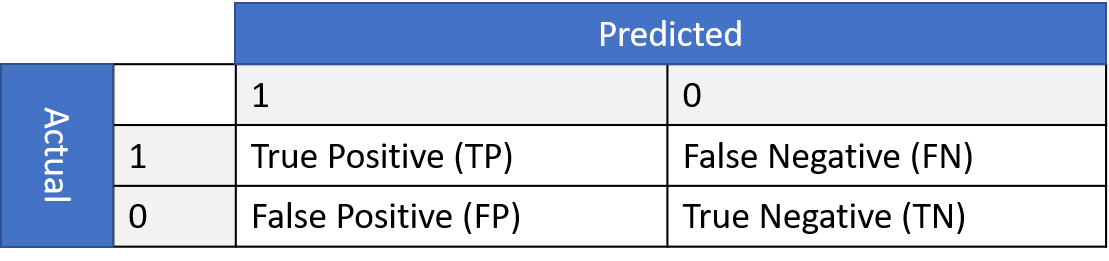Image by author

TPR = TP/(TP+FN)

FPR = FP/(TN+FP)

TPR or True Positive Rate answers the question — When the actual classification is positive, how often does the classifier predict positive?

FPR or False Positive Rate answers the qestion — When the actual classification is negative, how often does the classifier incorrectly predict positive?

To understand it more clearly, let us take an example of the current COVID situation. Assume that we have data for COVID patients and using some classifier we were able to classify the patients as positive and negative.

Let us now, without going into further details have a look at the distribution of the predicted classes. Here, again for simplicity let us assume that the data is balanced i.e. negative and positive classes are almost equal, additionaly they follow a normal distribution.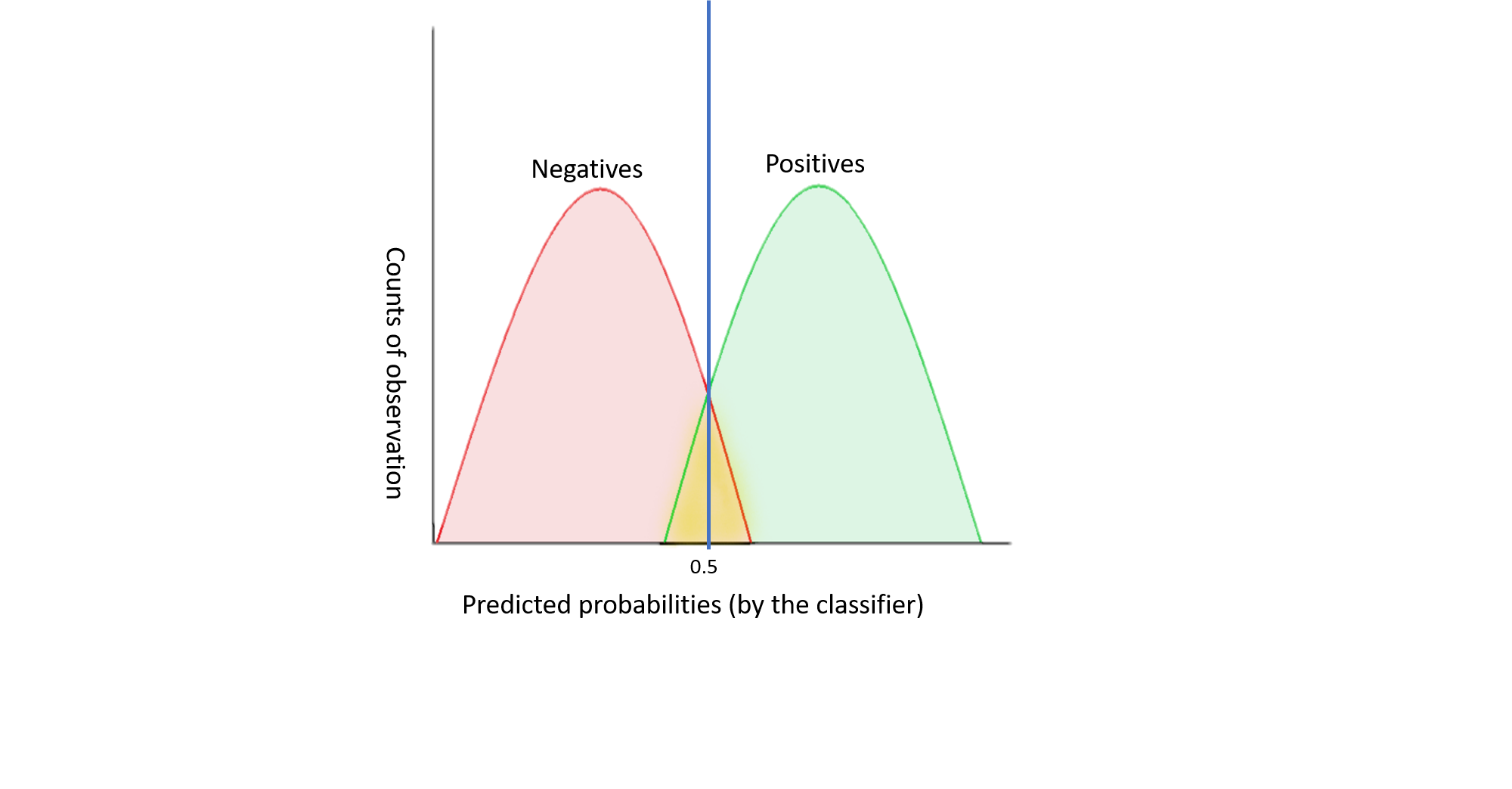Image by author

In the above graph, my classifier is doing a great job in classifying the patients — positive and negative. If I calculate the accuracy for such model, it will be quite high. Now, for different values of threshold, I can go ahead and calculate my TPR and FPR. According to the graph let us assume, that my threshold =0.5. At this threshold, the number of patients for which my classifier predicted a probability of 0.5, half were negative and half were positive.Similarly, I can check for other thresholds as well. For every threshold, TPR would be all patients in green area in the right of the threshold line divided by total patients in the green area.

FPR would be all patients in pink area in the right of the threshold line divided by total patients in the pink area.

## ROC Curve

Now, if I plot this data on a graph, I will get a ROC curve.

The ROC curve is the graph plotted with TPR on y-axis and FPR on x-axis for all possible threshold. Both TPR and FPR vary from 0 to 1.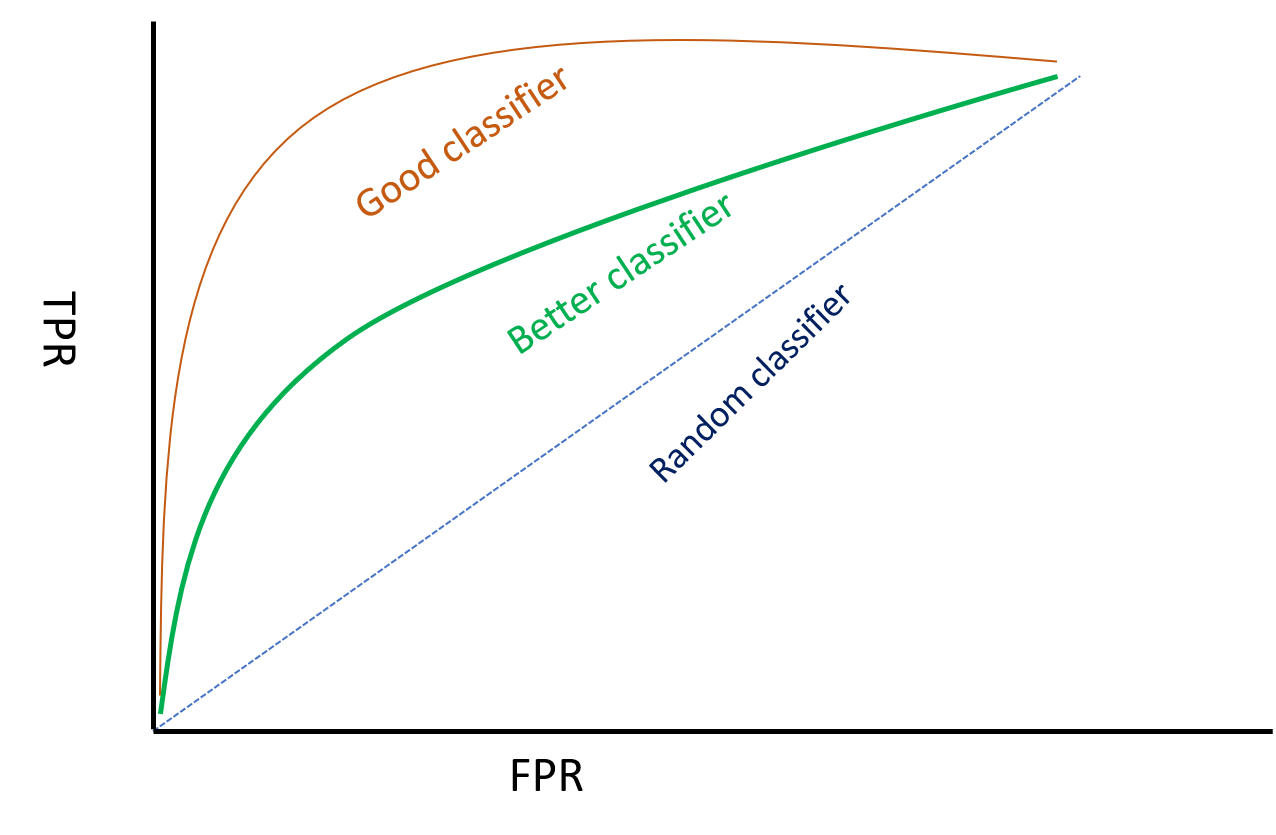Therefore, a good classifier will have an arc/ curve and will be further away from the random classifier line.

To qantify a good classifier from a bad one using a ROC curve, is done by AUC (Area under Curve). From the graph it is quite clear that a good classifier will have AUC higher than a bad classifier as the area under curve will be higher for the former.

From the above discussion, it is evident that ROC is a robust evaluation metrics than say Accuracy or Missclassification error because ROC takes into account all possible threshold levels whereas a metric like missclassification error takes only one threshold level into account.

The choice of your threshold depends on the business problem or domain knowledge. In our COVID patients example above, I would be okay with high FPR thus keeping my threshold levels low to ensure maximum COVID patients tracked.

#r #auc-roc #r-package #data-science #roc #data analysis1594839480

## In this post, I clearly explain what a ROC curve is and how to read it. I use a COVID-19 example to make my point and I also speak about the confusion matrix. Finally, I provide Python code for plotting the ROC and confusion matrix from multi-class classification cases.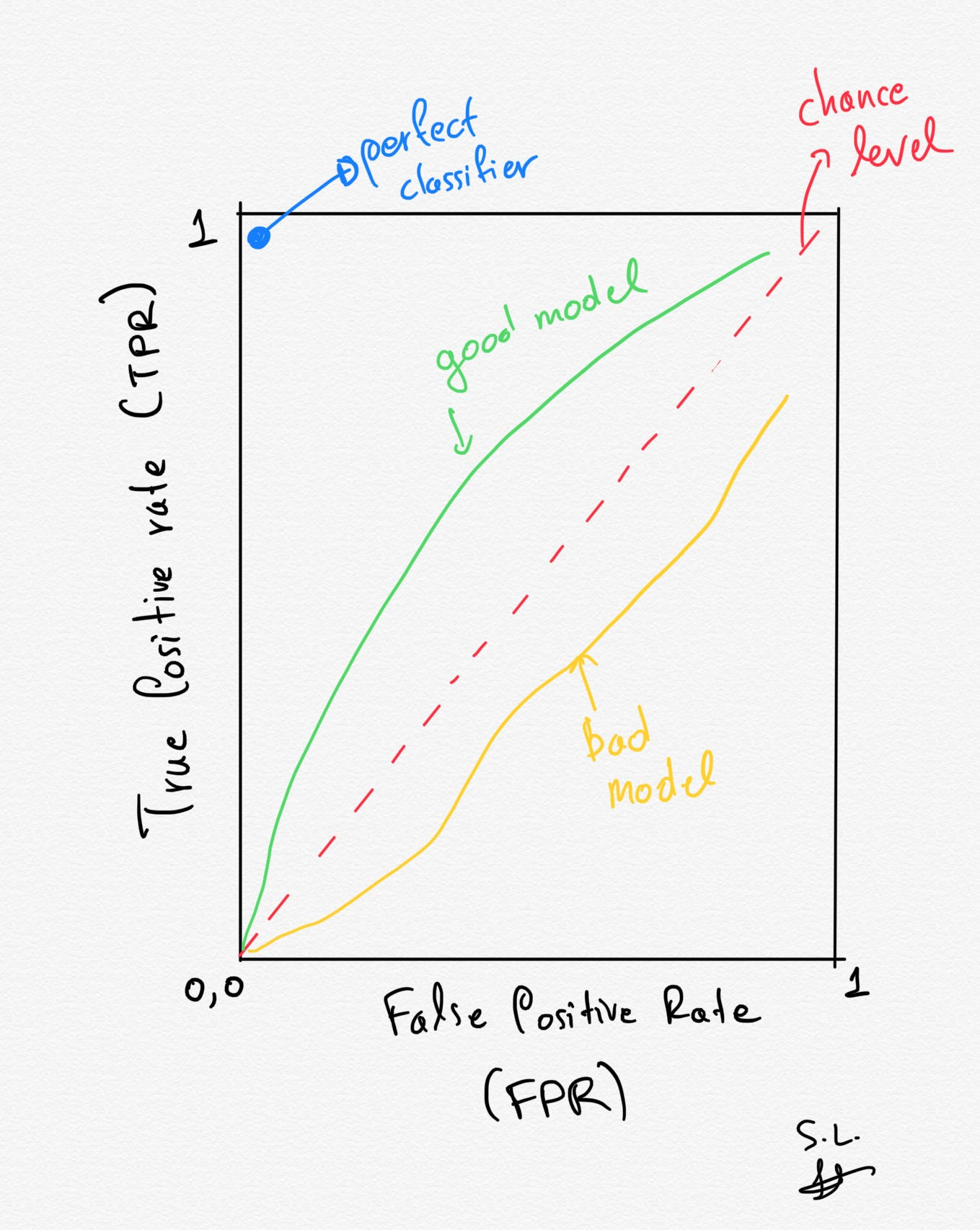# 1. Introduction

In 99% of the cases where a machine learning classification model is used, people report its ROC curve plot (as well as the AUC: area under the ROC) along with other metrics such as the accuracy of the model or the confusion matrix.

But what is a ROC curveWhat does it tell usWhy everyone is using them?** How is it connected to the confusion matrix?** Continue reading and you will be able to answer all these questions.

# 1.1. ROC Definition

A **receiver operating characteristic curve (ROC) curve **is a plot that shows the diagnostic ability of a binary classifier as its discrimination threshold is varied.

Before I dig into the details, we need to understand that this discrimination threshold is not the same across different models but instead it is model-specific. For instance, if we have a Support Vector Machine (SVC) then this threshold is nothing more than the bias term of the decision boundary equation. By varying the bias in a SVM model, we actually just change the position of the decision boundary. Have a look at my previously published SVM article for more details about the SVM models.

The ROC curve is created by plotting the true positive rate (TPR) against the false positive rate (FPR) at various threshold settings. The true-positive rate is also known as sensitivityrecall or probability of detection in machine learning. The false-positive rate is also known as the probability of false alarm and can be calculated as (1−specificity). It tells us how well our model can distinguish the classes.

A lot of terminology, right? Hold on a second, I will explain all these terms in the next section using an example that will make you always remember all these terms.

# 1.2. Terminology clearly explained (TP, TN, FP, FN)

## The COVID-19 test example

Let’s imagine that we have a** COVID-19 test **that is able within seconds to tell us if one individual is affected by the virus or not. So the output of the test can be either Positive (affected) or Negative (not affected) — we have a binary classification case.

Let’s also suppose that we know the ground truth and that we have 2 populations:

• a) people that are really affected (TP: True Positives, blue distribution in the figure below) and
• b) people that are **not affected **(TNTrue Negatives, red distribution in the figure below) — binary classification case.

#auc #roc #classification #machine-learning #data-science #data analysis1649300890

## ROC and AUC | How to Create and Interpret ROC Graphs Step-By-Step

ROC (Receiver Operator Characteristic) graphs and AUC (the area under the curve), are useful for consolidating the information from a ton of confusion matrices into a single, easy to interpret graph. This video walks you through how to create and interpret ROC graphs step-by-step. We then show how the AUC can be used to compare classification methods and, lastly, we talk about what to do when your data isn't as warm and fuzzy as it should be.

0:00 Awesome song and introduction
0:48 Classifying samples with logistic regression
4:03 Creating a confusion matrices for different thresholds
7:12 ROC is an alternative to tons of confusion matrices
13:44 AUC to compare different models
14:28 False Positive Rate vs Precision
15:38 Summary of concepts

#statquest #ROC #AUC #calculator #machinelearning1625776440

## ROC Curve and AUC. Simple Explanation (with Example)

ROC and AUC curves with simple example are explained at this video which dedicated to all data scientists, and to all Machine Learning specialists.

Explanation is divided into following parts:

• How do we use prediction threshold (cut-off line).
• How do we plot data on Logistic Regression (Sigmoid) curve
• How do we understand Confusion Matrixes (True Positives, True Negatives, False Negatives, and False Positives), Supervised Learning approach.
• How we calculate TPR (True Positive Rate) and how do we use it in plotting ROC.
• How we calculate FPR (False Positive Rate) and how do we use it in plotting ROC (Receiver operating characteristic).
• How we draw AUC (Area Under the Curve) based on ROC curves.
• How we can compare different Machine Learning Binary Classification models and algorithms with AUC.

For get benefit of this video you do not need to know Python or R. Just be careful about main definition of statistics, calculus and go ahead.

I hoped to create one of the best explainable video on Youtube about ROC and AUC. Comment bellow and drop your opinion!

#roccurve
#auccurve
#machinelearningtutorial

#roccurve #auccurve #machinelearningtutorial1627450200

## Laravel AJAX CRUD Example Tutorial

Hello Guys,

Today I will show you how to create laravel AJAX CRUD example tutorial. In this tutorial we are implements ajax crud operation in laravel. Also perform insert, update, delete operation using ajax in laravel 6 and also you can use this ajax crud operation in laravel 6, laravel 7. In ajax crud operation we display records in datatable.

### https://techsolutionstuff.com/post/laravel-6-crud-tutorial-with-example

#laravel ajax crud example tutorial #ajax crud example in laravel #laravel crud example #laravel crud example with ajax #laravel #php